# [3.NBT.3-1.0] Intro multiplication - Common Core Standard

##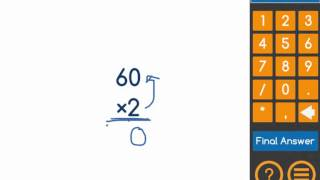By Freckle education

Multiply one-digit whole numbers by multiples of 10 in the range 10–90# Multiplying by multiples of 10, 100, and 1,000.

##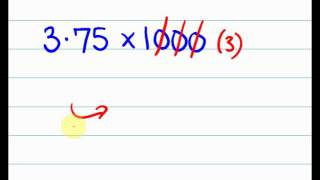By tecmath# MAFS.3.NBT.1.3

##By Brittany Bezada

We are multiplying one-digit whole numbers by multiples of 10 in the range 10-90 using strategies based on place value and properties of operations.# ast Mental Multiplication Trick - multiply in your head using base 10

##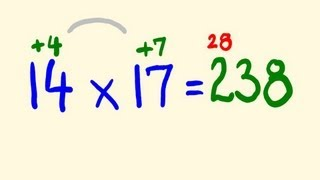By tecmath# Multiplying by 10

##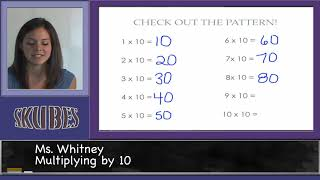By Skubes ed

Multiply one-digit whole numbers by multiples of 10 in the range 10–90 (e.g., 9 × 80, 5 × 60) using strategies based on place value and properties of operations.# [3.NBT.3-1.0] Intro multiplication - Common Core Standard

##By Freckle by Renaissance

Multiply one-digit whole numbers by multiples of 10 in the range 10–90 (e.g., 9 × 80, 5 × 60) using strategies based on place value and properties of operations. Front Row is a free, adaptive, Common Core aligned math program for teachers and students in kindergarten through eighth grade. Front Row allows students to practice math at their own pace - learning advanced concepts when they're ready and receiving remediation when they struggle. Front Row provides teachers with access to a detailed data dashboard and weekly email reports that show which standards are causing students difficulty, what small groups can be formed for interventions, and how their students are progressing in math.# One-step equations with multiplication and division

##By Khan Academy

Let's get a conceptual understanding of why one needs to divide both sides of an equation to solve for a variable.# One-step equations with multiplication and division

##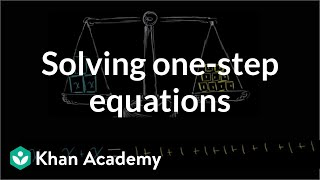By Khan Academy

This equation can be simplified through a single step to solve for the variable. Can you help?# One-step equations with multiplication and division

##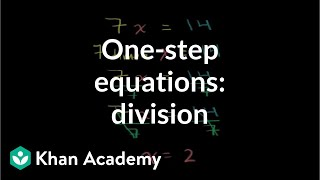By Khan Academy

Let's ease into this, shall we? Here's an introduction to basic algebraic equations of the form ax=b. Remember that you can check to see if you have the right answer by substituting it for the variable!# One-step equations with multiplication and division

##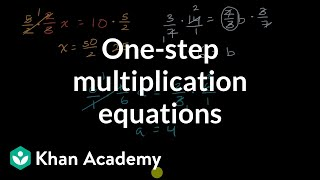By Khan Academy

Remember that what you do to one side, you have to do to the other. Will you multiply or divide both sides to dump the fraction, x/a? Let's do it together.# Dividing Fractions | MathHelp.com

##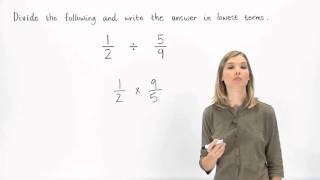By MathHelp.com# Grade 5 Math - Multiply Decimals 1

##By Lumos Learning

Using the Lumos Study Programs, parents and educators can reinforce the classroom learning experience for children and help them succeed at school and on the standardized tests. Lumos books, dvd, eLearning and tutoring are used by leading schools, libraries and thousands of parents to supplement classroom learning and improve student achievement in the standardized tests.# What is an Exponent & Powers of 10? - 

##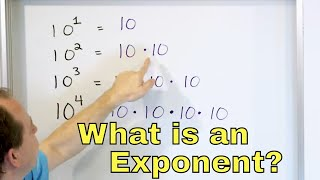By Math and Science

Quality Math And Science Videos that feature step-by-step example problems!# Grade 3 Math - Multiplication and Division Properties 1

##By Lumos Learning

Using the Lumos Study Programs, parents and educators can reinforce the classroom learning experience for children and help them succeed at school and on the standardized tests. Lumos books, dvd, eLearning and tutoring are used by leading schools, libraries and thousands of parents to supplement classroom learning and improve student achievement in the standardized tests.# Fraction Word Problems | MathHelp.com

##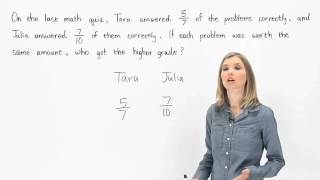By MathHelp.com# One-step equations with multiplication and division

##By Khan Academy

Let's ease into this, shall we? Here's an introduction to basic algebraic equations of the form ax=b. Remember that you can check to see if you have the right answer by substituting it for the variable!# One-step equations with multiplication and division

##By Khan Academy

Remember that what you do to one side, you have to do to the other. Will you multiply or divide both sides to dump the fraction, x/a? Let's do it together.# One-step equations with multiplication and division

##By Khan Academy

This equation can be simplified through a single step to solve for the variable. Can you help?# One-step equations with multiplication and division

##By Khan Academy

Let's get a conceptual understanding of why one needs to divide both sides of an equation to solve for a variable.# [3.OA.5-1.0] Multiplication Properties - Common Core Standard

##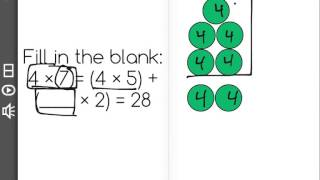By Front Row

Discover more Common Core Math at https://www.frontrowed.comApply properties of operations as strategies to multiply.2 Examples: If 6 Ãƒâ€” 4 = 24 is known, then 4 Ãƒâ€” 6 = 24 is also known. (Commutative property of multiplication.) 3 Ãƒâ€” 5 Ãƒâ€” 2 can be found by 3 Ãƒâ€” 5 = 15, then 15 Ãƒâ€” 2 = 30, or by 5 Ãƒâ€” 2 = 10, then 3 Ãƒâ€” 10 = 30. (Associative property of multiplication.) Knowing that 8 Ãƒâ€” 5 = 40 and 8 Ãƒâ€” 2 = 16, one can find 8 Ãƒâ€” 7 as 8 Ãƒâ€” (5 2) = (8 Ãƒâ€” 5) (8 Ãƒâ€” 2) = 40 16 = 56. (Distributive property.)Front Row is a free, adaptive, Common Core aligned math program for teachers and students in kindergarten through eighth grade. Front Row allows students to practice math at their own pace - learning advanced concepts when they 're ready and receiving remediation when they struggle. Front Row provides teachers with access to a detailed data dashboard and weekly email reports that show which standards are causing students difficulty, what small groups can be formed for interventions, and how their students are progressing in math.Discover more Common Core Math at https://www.frontrowed.com